####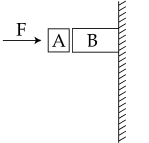Given in the figure are two blocks A and B of weight 20 N and 100 N, respectively. These are being pressed against a wall by a force F as shown.  If the coefficient of friction between the blocks is 0.1 and between block B and the wall is 0.15, the frictional force applied by the wall on block B is : Option 1) 100 N Option 2) 80 N Option 3) 120 N Option 4) 150 N

As we learnt in

Motion of Block in Contact -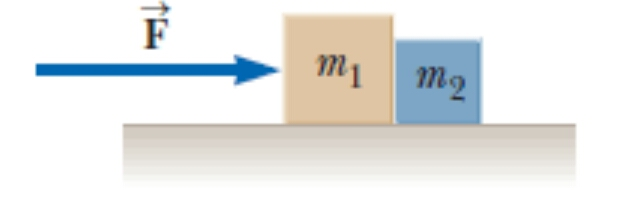- whereinStatic Friction -

Self adjusting force because it changes itself according with the applied force.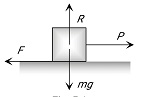- wherein

It always equal to net external force. Static friction (F = P) using diag.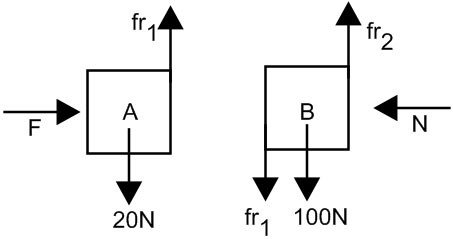N = F

fr1 = 20 N

fr2 = 100 + 20 = 120 N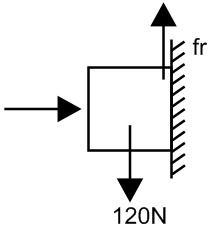Where assume two blocks as one system due to equilibrium.

fr =120 N

Correct option is 3.

Option 1)

100 N

This is an incorrect option.

Option 2)

80 N

This is an incorrect option.

Option 3)

120 N

This is the correct option.

Option 4)

150 N

This is an incorrect option.

#### Vakul